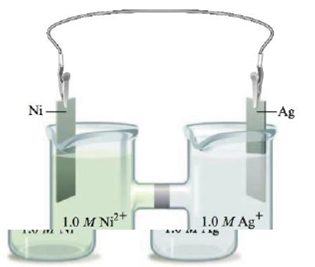Chapter 18, Problem 151CP

Chapter
Section
Textbook Problem

# Consider the following galvanic cell:Calculate the concentrations of Ag+(aq) and Ni2+(aq) once the cell is “dead.”

Interpretation Introduction

Interpretation:

The set up of a galvanic cell having Silver and Nickel electrode is given. The concentration of Ag+(aq) and Ni2+(aq) once the given cell is dead is to be calculated.

Concept introduction:

The equilibrium constant is used to determine the direction of the equilibrium. The higher value of K corresponds to the forward reaction and the lower value of K corresponds to the backward reaction.

To determine: The concentration of Ag+(aq) and Ni2+(aq) once the given cell is dead.

The value of K for the given cell reaction is 7.24×1034_ .

Explanation

The reaction taking place at the cathode is,

Ag++eAgE°red=0.80V

The reaction taking place at the anode is,

NiNi2++2eE°ox=0.23V

Multiply the reduction half-reaction with a coefficient of 2 and then add both the reduction and oxidation half-reactions as,

2Ag++2e2AgNiNi2++2e

The final equation is,

Ni+2Ag+2Ag+Ni2+

The value of E°cell is given as,

cell=E°ox+E°red

Where,

• ox is the oxidation potential of the electrode.
• red is the reduction potential of the electrode.

Substitute the values of E°ox and E°red in the above equation as,

cell=E°ox+E°red=0.23V+0.80V=1.03V

The cell potential is calculated using the Nernst equation,

E=E°-(0.0591n)log(Q)

Where,

• E is the cell potential.
• is the cell potential at the standard conditions.
• Q is the activity of the species in the cell.
• n is the number of electrons involved in the reaction.

As the cell is dead, it means the reaction has reached equilibrium. Therefore, at equilibrium, the value of Q is replaced with the equilibrium constant K and at equilibrium the value of E is equal to zero.

The reaction involves the transfer of 2 moles of electrons.

Rearrange the equation to obtain the value of E° as,

E°=E-(0.0591n)log(K)

Substitute the values of E , E° , n , in the above equation as,

E=E°-(0.0591n)log(K)0-1.03=-(0.05912)log(K)log(K)=34.86K=1034.86

The equation is further simplified as,

K=1034.86=7.24×1034_

The value of K for the given cell reaction is 7.24×1034_ .

The concentration of Ag+ from the reverse reaction is 0.46×10-17M_ and the concentration of Ni2+ from the reverse reaction is 1.5M_ .

The value of K for the given cell reaction is 7.24×1034_ .

As the value of equilibrium constant is high, it means that the reaction is about to complete.

Make the ICE table for the given cell reaction,

2Ag++Ni2Ag+Ni2+Initial:1

### Still sussing out bartleby?

Check out a sample textbook solution.

See a sample solution

#### The Solution to Your Study Problems

Bartleby provides explanations to thousands of textbook problems written by our experts, many with advanced degrees!

Get Started

#### Find more solutions based on key concepts